# Hint: Voltage is the same in both cases There is a single loop circuit that has...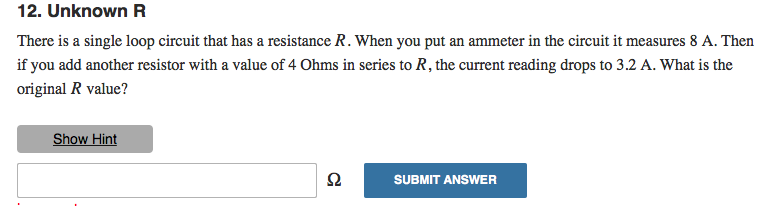Hint: Voltage is the same in both cases

There is a single loop circuit that has a resistance R. When you put an ammeter in the circuit it measures 8 A. Then if you add another resistor with a value of 4 Ohms in series to R, the current reading drops to 3.2 A. What is the original R value?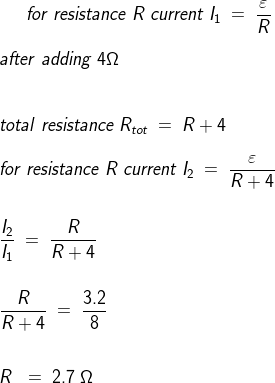#### Earn Coin

Coins can be redeemed for fabulous gifts.

Similar Homework Help Questions
• ### A single-loop circuit consists of a 9.0 V battery and a 22 Ω resistor. How much...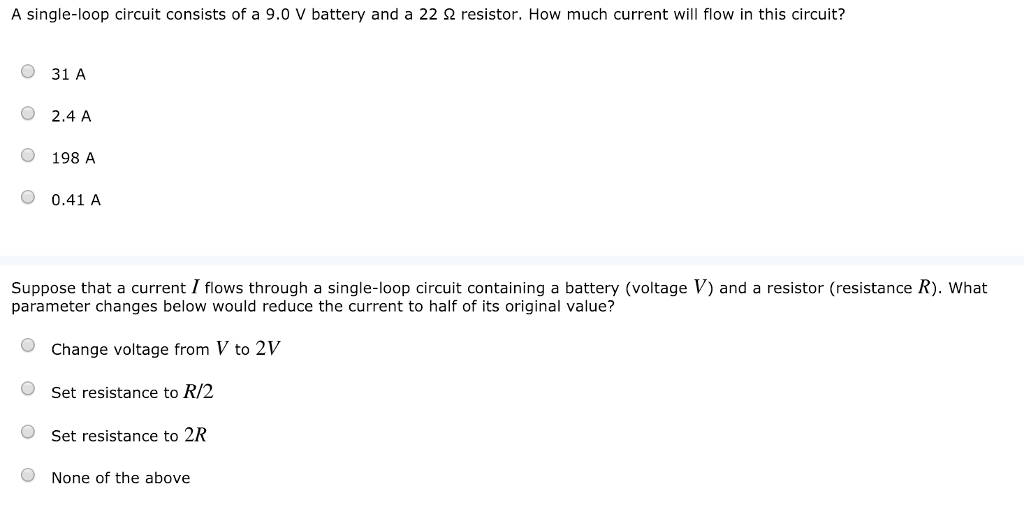A single-loop circuit consists of a 9.0 V battery and a 22 Ω resistor. How much current will flow in this circuit? O 31 A O 2.4 A O 198 A O 0.41 A Suppose that a current I flows through a single-loop circuit containing a battery (voltage V) and a resistor (resistance R). What parameter changes below would reduce the current to half of its original value? OChange voltage from V to 2V OSet resistance to R/2 O Set...

• ### In this circuit, three resistors receive the same amount of voltage (24 volts) from a single...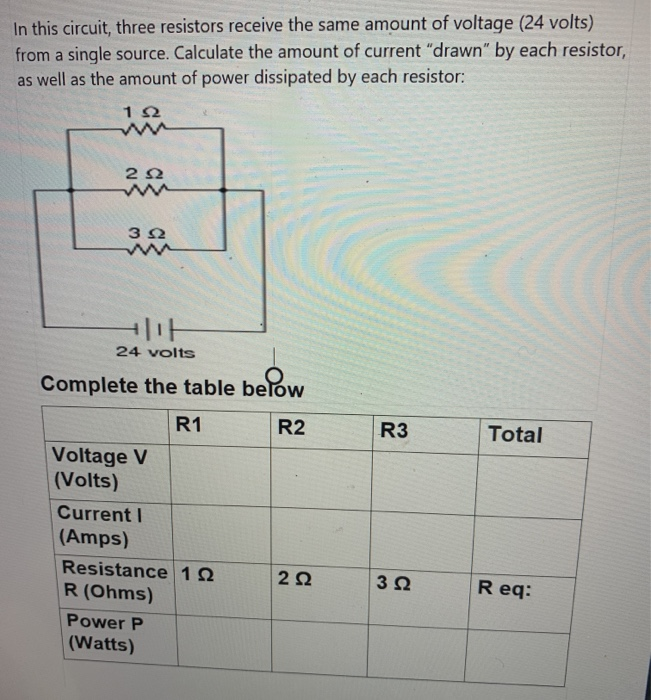In this circuit, three resistors receive the same amount of voltage (24 volts) from a single source. Calculate the amount of current "drawn" by each resistor, as well as the amount of power dissipated by each resistor: 12 2 12 3 Ω w 24 volts Complete the table below below R1 R2 R3 Total Voltage V (Volts) Current (Amps) Resistance 1.22 R (Ohms) Power P (Watts) 2 Ω 322 Req:

• ### Question 2 (5 marks) In a single-loop LRC-series circuit, Kirchhoffs second law states that the sum of voltage drop...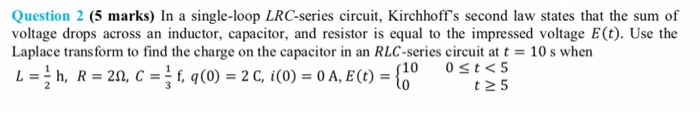Question 2 (5 marks) In a single-loop LRC-series circuit, Kirchhoffs second law states that the sum of voltage drops across an inductor, capacitor, and resistor is equal to the impressed voltage E(t). Use the Laplace trans form to find the charge on the capacitor in an RLC-series circuit at t 10 s when Lh, R 20, C = f, q(0) = 2 C, i (0) = 0 A, E(t) (10 0 st<5 t 5 Question 2 (5 marks) In a...

• ### The current in a single-loop circuit with one resistance R is 7.3 A. When an additional...

The current in a single-loop circuit with one resistance R is 7.3 A. When an additional resistance of 2.2 Ω is inserted in series with R, the current drops to 5.9 A. What is R?

• ### The current in a single-loop circuit with one resistance R is 7.2 A. When an additional...

The current in a single-loop circuit with one resistance R is 7.2 A. When an additional resistance of 3.0 Ω is inserted in series with R, the current drops to 4.7 A. What is R?

• ### Draw a circuit with a resistance(R=V/I) of 1500 ohms, but you only have access to 1...

Draw a circuit with a resistance(R=V/I) of 1500 ohms, but you only have access to 1 resistor of 1000 ohm. Hint: use exactly 3 resistors. Use wires, switches, battery, voltmeter, and ammeter, regular resistors of 10 ohms. You can change your resistor value. Do this by using equivalent resistance equations for both series and parallel.

• ### Question One: In the figure below, what is the reading on the ammeter in each case...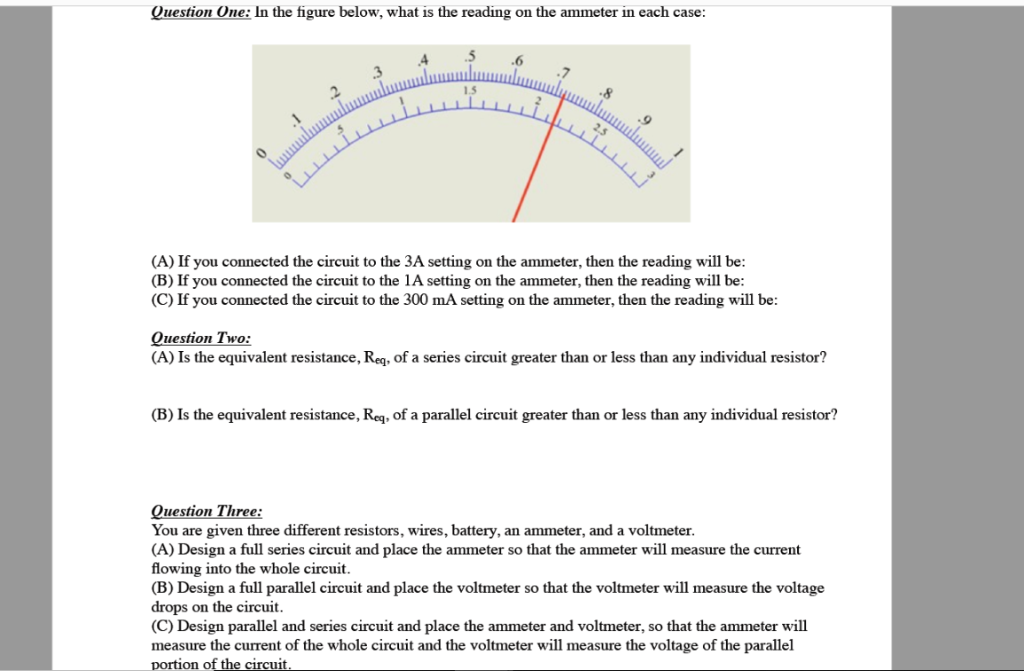Question One: In the figure below, what is the reading on the ammeter in each case .5 .6 (A) If you connected the circuit to the 3A setting on the ammeter, then the reading will be (B) If you connected the circuit to the 1A setting on the ammeter, then the reading will be (C) If you connected the circuit to the 300 mA setting on the ammeter, then the reading will be uestion Two: (A) Is the equivalent resistance,...

• ### 3. If the circuit in Fig. 2 is built and operated, it will be found that the resistor becomes much hotter than the inductor, even though both components drop the exact same amount of voltage and carr...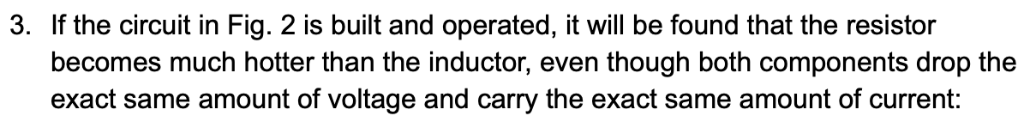3. If the circuit in Fig. 2 is built and operated, it will be found that the resistor becomes much hotter than the inductor, even though both components drop the exact same amount of voltage and carry the exact same amount of current: 339.4 Alternator 34 2 9.02 mH 480 VAC 60 Hz 3394 Fig. 2. Alternator driving a series loop with resistor and inductor. There is an identical voltage drop across each device, and they carry exactly the same...

• ### NAME RESISTORS IN SERIES CIRCUITS Construct the circuit Figure using The Circuit Construction KCK) simulation at...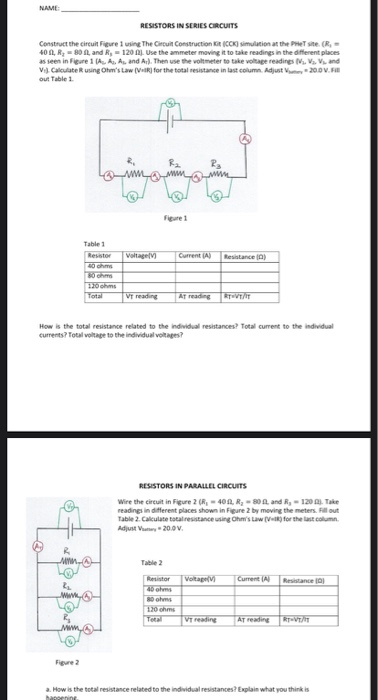NAME RESISTORS IN SERIES CIRCUITS Construct the circuit Figure using The Circuit Construction KCK) simulation at the Presite - 400, R; -90 and R, -120 . Use the ammeter moving it to take readings in the different places as seen in Figure 1A. A As and Ar). Then use the voltmeter to take voltage readings Ms. V. Vand V. Calculate Rusing Ohms Law (Vol for the total resistance in last column. Adjust-20.0V.FI out Table 1 www Table 1 Resistor Gm...

• ### Chapter 27, Problem 015 The current in a single-loop circuit with one resistance R is 4.9...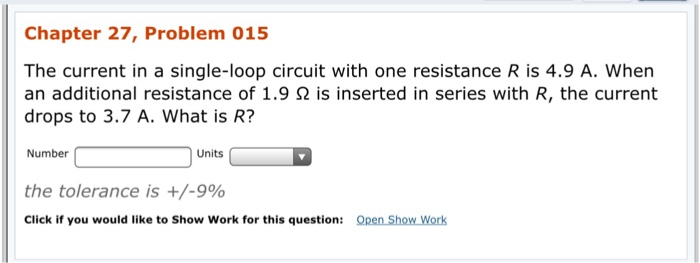Chapter 27, Problem 015 The current in a single-loop circuit with one resistance R is 4.9 A. When an additional resistance of 1.9 2 is inserted in series with R, the current drops to 3.7 A. What is R? Number Units Units the tolerance is +/-9% Click if you would like to Show Work for this question: Open Show Work

Free Homework App